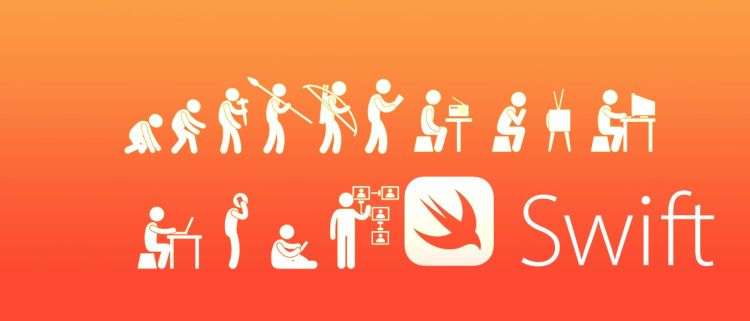← back to the blog

# Swift 5 / 5.1 Enumeration with enum and enum method example

##### Posted on November 23rd, 2019 in Swift by GeorgeCreate enumeration based on popular cards set Ranking.

``````enum Rank: Int {
case ace = 15
case two = 2, three = 3, four = 4, five = 5, six = 6 , seven = 7, eight = 8 , nine = 9, ten = 10;
case jack = 12 , queen = 13, king = 14

func compareValues(v:Rank) -> String {
if self.rawValue > v.rawValue {
return "\(self) has primacy";
}
return "\(v) has primacy";
}

func simpleDescription() -> String {
switch self {
case .ace:
return "ace"
case .jack:
return "jack"
case .queen:
return "queen"
case .king:
return "king"
default:
return String(self.rawValue)
}
}
}
let ace = Rank.ace;
let two = Rank.two;
let seven = Rank.seven;
let aceRawValue = ace.rawValue
print(seven.compareValues(v: two))

``````

Thank you.